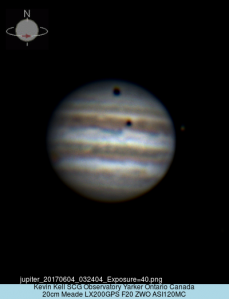True Field of View

In the past, when imaging Jupiter. I did a full field image run, put the image up on the screen, found out the apparent diameter of Jupiter and physically measured the screen and Jupiter to determine TFOV.

This past weekend, Kim & I tried it out on a star. Using firecapture and the reticle turned on, we centered the star, stopped the tracking and timed how log it took to hit an edge. Luckily for us, it went on a diagonal and exited almost exactly at the corner in 12.69 seconds.

* drift test for FOV wth ASI290MC camera, no reducer, no barlow, on the Torus telescope D=400mm F10, FL=4000mm
12.69 seconds from centre to top right corner exit.
camera pixel resolution=1936×1096.

The centre reticle location is 1/2 of that, so 548×978
Using right angle geometry, the diagonal is 1121 pixels, full diagonal would be twice that or 2242 pixels
calculate drift 1/2 FOV is 12.69 seconds
full FOV is 25.38 seconds

360 degrees / 24 hours = 15 degrees/hour
15 degrees/hr / 60 minutes/hr = 0.25 degrees/minute (15 arcmin/minute)
15 arcmin/minute / 60 seconds/minute = 0.25 minutes/second (15 arcseconds/second)
So just count how long it takes in seconds for a star to move across an eyepiece’s FOV and multiply it by 15 to get the FOV in arcseconds.
TFOV(diagonal)=15*Time
TFOC(diagonal)=15*25.38=381 arcseconds = 6 minutes 20 seconds diagonal

ZWOASIM290MC FOV 1936×1096 pixels gives 329 x 186 arc seconds rectangle

Turns out this method is WRONG! (*Unless* you are using a star with a declination of 0)

The True Field Of View (TFOV) is then:
TFOV = 15.04*T*Cos(delta)
where “delta” is the star’s declination, “Cos” is the Cosine function, and “T” is the measured drift time interval. If the time is measured in minutes, the true field will be in minutes of arc, and if the time is in seconds, the true field will be in seconds of arc. For example, if a star has a declination of 25.5 degrees (ie: 25 degrees 30 minutes), and a measured drift time of 2.75 minutes (ie: 2 minutes 45 seconds), the true field of view is then 37.3 arc minutes in diameter. For stars within 3 degrees of the celestial equator, the Cosine function can be approximated to 1, and the formula becomes:

We used a star near saturn (mag 0.52 38 arcseconds with rings)
HIP 93423 mag 6.2 dec -22deg 39min = 22.65 degrees
cosine 22.65 degrees is 0.923
TFOV=15.04*25.38seconds* 0.923= 352 arcseconds (less than original calculation of 381)

so ZWOASI290MC TFOV= diagonal 352 arcseconds, rectangle 303×172 arcseconds

Then someone mentioned you can do the same with just the focal length and the camera sensor chip size, which led to this
formula:

I found: sensor size (mm) * 135.3/focal length (inches) = arcminutes
mixing units seems to be just wrong, but perhaps the fudge factor of 135.3 accounts for that?

the camera sensor size is 5.6×3.2mm
focal length of 4000mm=157 inches
5.6*135.3/157= 4.8 arcminutes
3.2*135.3/157=2.8 arcminutes

drift solution:
ZWOASI290MC TFOV= diagonal 352 arcseconds, rectangle 303×172 arcseconds = 5×3 arcminutes

So the two answers are reasonably the same, within error limits.